## "Information Entropy for The Clinical Evaluation of Autism Spectrum Disorders"

Thiago Rafael da Silva Moura*

Facult of Sciences, Federal University of Pará, Interdisciplinary Innovation Laboratory, Raimundo Santana Street, Salinópolis - PA, 68721-000, Brazil

*Corresponding author: Thiago Rafael da Silva Moura, Facult of Sciences, Federal University of Pará, Interdisciplinary Innovation Laboratory, Raimundo Santana Street, Salinópolis - PA, 68721-000, Brazil. Tel: +5591983675044: Email: trsmoura@yahoo.com.br

Received Date: 29 May, 2018; Accepted Date: 15 June, 2018; Published Date: 21 June, 2018

# 1.       Abstract

The absence of biomarkers hinders the early diagnosis of children with Autism Spectrum Disorders (ASDs) applied in routine examinations in newborns, for example. At moments, post-birth years, difficulties in social interaction and other emerging factors are used for the evaluation of children with ASD. We have reported numerical results that analyze the social interaction and the repetitive behavior of children with and without ASD. To represent the interaction, by analogy, we used two sets of random walkers. These sets are labeled in two ways, a group, e.g., the first set of random walkers represents children with autism, characterized by persistent and change-resistant microscopic dynamics. The second set of walkers, which we labeled as healthy, is represented as a set that presents diffusive regimes, this group is represented by the Elephant Random Walks (ERW) model. We have analyzed, from the perspective of information entropy, how the two sets of random walkers influence the entropy variations of each other. Walkers interact in pairs; the impact of this interaction is analyzed in the entropy variations of each of the sets. Therefore, we have reported the entropy variations for the ERW model and its variations when the interaction becomes stronger with probability f. We have reported that, surprisingly, f changes do not provoke changes in entropy for the group of random walkers who represent the group of children with autism.

2.       Keywords: Autism; Entropy; Interaction; Invasive Disorder; Random Walks

1.       Introduction

We have reported the use of discrete random walks as a diagnostic tool for the analysis of developmental disorders. Developmental disorders are classified into two subtypes: specific disorder and pervasive disorder. Delays in development in one or more specific areas is a characteristic of developmental disorder. The other subtype, the pervasive disorder, presents deficiencies in basic functions in multiple contexts that include socialization and communication. Pervasive De- Velopmental Disorders (PDD’s) are part of the group of disorders of the Autistic Spectrum (ASD), known as neurodevelopmental disorder. Symptoms accompanying individuals with ASD are the delay in verbal and non-verbal communication; resistance to routine change; restricted and persistent interests in relation to an activity, topic, object, speech, idiosyncratic phrases, etc.; abnormalities in eye contact and body expression; difficulties in starting and maintaining social relations. Each of these symptoms may vary from mild, moderate or severe and are part of the diagnostic criteria of the autistic spectrum disorders used in routine examinations [1-3]. Inspired by these symptoms and the diagnostic criteria for ASDs, we constructed a model using discrete random walks as a diagnostic tool for autism. Our starting point is to define a model to compare with autistic behavior. The model we chose as the standard of comparison is the Elephant Random Walks Model (ERW). The ERW presents pertinent characteristics to be chosen as the standard model: their diffusive regimes are well known, the model is accurate and several variations have been proposed with the objective of exploring distinct search mechanisms in memory, the loss of in- formation and its impact on the diffusive process, among other characteristics [4-7]. Further details can be found in [8-15].

2.       The model

Our model consists of pairs of random walkers. The ﬁrst walker, we call” Professor”; the second one,” Student”. We consider that in neurological exams in children the analysis of communication and social interaction in multiple contexts is part of the routine, including repetitive patterns of behavior, the examiner (physician) has knowledge of the patient’s actions; therefore, in the context of our problem, the Professor has knowledge of the student’s actions, but he/she is not inﬂuenced by these actions. In this case, by analogy, Physician is the Professor and the patient is the Student. However, what happens in a context where the Professor is also inﬂuenced by the Student? This problem can be analyzed as follows: The Professor can learn (not learn) from Student’s actions with probability fP (1-fP), similarly, the Student can learn (not learn) with the Professor probability fA (1-fA). Random walker’s pairs can learn from each other, both can learn Professor and Student labels. For reasons of order, let’s call the ERW model “Professor” and the other one “Student”.

2.1.  The Professor

We deﬁned the” Professor” as the ERW model. Nevertheless, we have described the relevant aspects of Schu¨tz and Trimper, their main characteristics and diﬀusion regimes. The model of random walks with uniform memory proﬁle considers a discrete random walk so that each decision, taken at every moment of time, depends on the spectrum of decisions taken in every previous history of the walker in an equitable manner. Recovering each past decision in an equable manner is a characteristic of a random walk with uniform memory proﬁle. The probability that an action taken in the past will be remembered is 1/t, to t the current time. The random walk is recorded at all times, this aspect attributes to the process a non-Markovian characteristic. The stochastic dynamics of the process occur in the following way: the walker starts in time t0 in a position x0, at each instant of time the walker walks one step to the right or step to the left, with probability p and (1-p), respectively. For this process, the stochastic evolution equation is given by

Xt+1 = Xt + σt+1.                                                                                                                                          (1)

for the time t + 1. The variable σt+1 assumes the value +1 when the walker walks one step to the right and -1 when the walker walks one step to the left. The memory consists of a set of random variables σtj for the time t prime < t   that the walker can recover. This process occurs as follows:

(a)                 in time t+1 a number t0 of the set 1,2,..,t is chosen randomly with uniform probability 1/t.

(b)                 σt+1 is determined stochastically by following the following relations, σt+1 = σt0 with probability p and σt+1 = -σt0 with probability 1-p.

The ﬁrst step at time t = 1, is taken according to the following dynamics: the walker is in the position X0 and moves to the right with probability q or to the left with probability 1-q, i.e., σ1 = +1 with probability q and σ1 = -1 with probability 1-q. The stochastic evolution equation is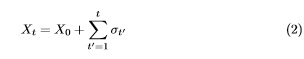The parameter p is the probability of the walker repeating an action from the past in a time tj. When (p > 1/2) the walker presents a persistent behavior, this is characterized by the repetition of past actions. For (p < 1/2) the walker takes an action contrary to the action that was selected, presenting an anti- persistent behavior. For the value of (p = 1/2) the random walk is Markovian. In the boundary regions of (p = 0) and (p = 1) two extreme behaviors arise. In (p = 0) the maximum of the anti-persistent behavior occurs, while in (p = 1) the maximum persistent behavior occurs; in the latter case, the movement is ballistic, characterized by the typical value of the Hurst exponent (H = 1) . The diffusive behavior changes with the probability values p, when (p < 3/4) the second moment depends linearly on t and diffusion is ordinary (H = 1/2), para (p > 3/4) diffusion is characterized as super diffusive, the point (p = 3/4) separates the diffusive and super diffusive regimes. Additional information about the ERW model, such as the study of its limits and other models that are variants of it, are found in [7,16,17].

4.2   The Student

The microscopic dynamics of the student occurs in a manner similar to that of the Professor. The Student initiates his/her movement at the position Y0, at time t0 = 0, at every instant of time the random walker walks one step to the left or to the right. The stochastic equation that quantifies Student’s steps is given by

Yt+1 = Y0 + vt+1                                                             (3)

vt+1 is a random variable that assumes the values of σtt when the random walker behaves like the ERW model. The Student accepts the decisions of the ERW model with probability fA and rejects with probability (1 fA), when the walker makes decisions based on his/her own history. Memory is formed by a set of random variables vtt with tj < t, that the walker remembers the following way:

(a)in time t + 1 a number tj of the set 1, 2, ..., t is chosen in an equiprobable way 1/t.

(b)the variable vt+1 is determined stochastically as vt+1 = vtt .

At the moment t = 1, the walker is in position Y0 accepting (rejecting) the decisions of the ERW model with probability fA (1 fA), with the stochastic evolution equation quantifying this process for all time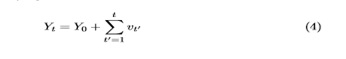4.3   The Professor and Student Interaction

The core of our model is to describe the interaction between random walkers and their consequences. The Professor can learn from the student’s microscopic decisions. Therefore, the Professor’s microscopic dynamics will be influenced by the Student with probability fP and not influenced with probability 1 fP. The stochastic equation describing this process is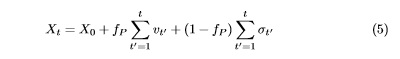The probability values fP = 0 and fP = 1 quantify the minimum and maximum learning capacity of the Professor, respectively. At least learning, the random walker behaves like the ERW model. In fP = 1, the Professor’s maximum learning in relation to the student’s actions occurs, quantified by the following stochastic relation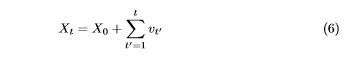and vtt is the stochastic variable that represents the microscopic decisions in Student.

The Student’s learning, in relation to the Professor’s microscopic dynamics, occurs as follows: The Student accepts (rejects) the Professor’s decisions with probability fA (1 fA). Therefore, the equation describing the stochastic evolution of the Student position is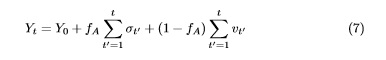For the maximum non-learning limit, fA = 0, the Student recalls actions solely from its history, its stochastic equation is given by (4), another extreme, fA = 1, when there is maximum interaction, the stochastic equation is written as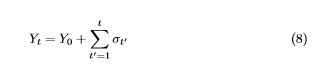The characteristic described above, which deals with the quantification of learning of random walkers, we defined as the process of Professor-Student interaction.

There are two cases for the learning consideration of Professor-Student interaction: the symmetric case and the non-symmetrical case. In the symmetrical case, the Professor and Student present the same probability of learning (fP = fA), in the non-symmetrical case, the Professor or Student presents a higher probability of learning than the other. Therefore, (fP > fA) when the Professor learns more easily from Student’s actions, (fP < fA) otherwise.

Let’s discuss the case in which (fP = fA). Therefore, we can vary a single value of probability, calling fP = fA = f, we can rewrite the equations (7) and (5), respectively as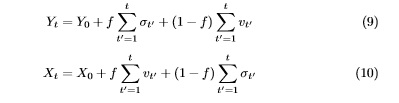For the case of minimal learning, f = 0, the models recover their ordinary results without mutual learning, with stochastic equations (4) and (2) for the Student and for the ERW model, respectively. The maximum learning occurs when f = 1, at this point, Professor and Student are at the maximum of mutual influence.

# 3.       Results

We analyze the variation of the Shannon entropy in the Professor-Student interaction process. The Shannon entropy, also known as information entropy, was formulated by Claude Shannon in a context different from what we are applying, in the context of telecommunications applications to analyze the economy expectancy related to the statistical structure in sending and receiving information . We will use Shannon’s entropy in the context of discrete random walks for the problems of Professor-Student interaction. The interaction depends on two control parameters: the probability of interaction between walkers f and the probability of the random walker p to repeat an action from the past in an instant tj. The information entropy associated with the diffusion of walkers is given by equation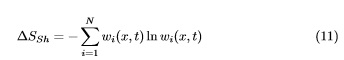And SSh is the Shannon entropy and wi (x, t) the probability of finding the system in the state i. With the variation in the parameters f and p, we have obtained a surface with several quantitative values of the entropy variation, each point accounts for a variation in the entropy for the diffusive process according to the variances of f and p. In order to normalize and improve the presentation of results, we have divided all the typical entropy values by the greater entropy variation found on the surface, max{S} = ∆Smax, we divided the equation 11 by Smax, therefore, it follows that.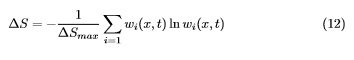S provides the variation of relative entropy associated with the diffusion process. The highest value of entropy, according to equation 12, is ∆S = 1, that occurs when ∆SShannon =SmaxFor the other values ∆S < 1We did not work with diffusion regimes, we focused on the interaction and their impact on the entropy variations of random walkers. The entropy for the set of random walkers labeled Student, according to the measurements obtained from the numerical simulations, is invariant under the changes of the probabilities p and f in the ranges 0 ™ p ™ 1 and 0 ™ f ™ 1. Therefore, for random walks of finite size, in the context of Professor-Student interaction, the variations in the parameters p and f do not imply in variations in the entropy, which is constant and equal to zero, S = 0.

For the Professor, the variations in the control parameters exert influence under the entropy variations. We will focus on the proper description of entropy for the Professor. We measured the entropy using the controllable parameters p and f , its variations allow us to quantify the entropy variations related to the ERW model, which was the model observed for the construction of ours, as well as the obtaining of quantitative values of the entropy that differ substantially

from those observed for the ERW  model.  In (Figure 1) the resulting diagram   of Professor-Student interaction in the representation of information entropy is presented. We present our results at the point f = 0, at the point of zero inter- action, in which there is no Professor-Student interaction, the typical entropy variations in the ERW model are displayed. At this point, f = 0, controlling the parameter p in the range 0 ™ p ™ 1, the entropy variations are approximately the same in the range 0 p 3/4, above p > 3/4 the variance of the entropy associated with the ERW model is larger the greater the values of p. In the range 0 ™ f ™ 0.1, there has been a rapid growth of entropy followed by a smoother decay in the range 0.1 < f “ 0.6. Approximately in the curve f   0.6, of all the curves in which f = 0, is the one with the smallest entropy variation with the lowest entropy variation of the point f “ 0.6 and p = 0. The greatest entropy variations occur in the curves where, first, p = 1 and 0 f 1; secondly, when we consider the maximum interaction curve between the random walkers f = 1 and 0 ™ p ™ 1, the greatest entropy variation occurs at the point p = 1 and    f = 1 of the surface.

(Figure 2) is a color map of   Figure 1. The variation of the intensity of the colors is related to the entropy variations, the regions with red tonalities are associated with larger entropy variations, the darker tones are related to smaller variations. We emphasize, on the map, the curve in which f = 1 of greater interaction between random walkers, accompanied by greater entropy variations, ∆S = 1. Contour curves are drawn by connecting points on the surface at which the entropy is invariant. We highlighted, centered on the left side of the Figure 2, the contour with entropy variation ∆S = 0.6, immediately above, to the extent that p grows, the curve with ∆S = 0.8, the entropy varies more strongly. Just below the curve with ∆S = 0.6, in the direction f decreases, we highlighted the curve of equal entropy with typical measurement ∆S = 0.4.

Another area that also should be highlighted is the area 0 p 1 and 0 f ™ 0.1. In Figure 3 we highlighted the entropy variations in the ERW model, f = 0, its variations in the extent that f grows. For this value of the interaction parameter, f = 0, the entropy variations are greater the greater they are values of the parameter p. Even though it is accompanied by a rapid variation of entropy, this region, 0 p 1 and 0 f 0.1, has a surface of equal entropy ∆S = 0.4, highlighted in the figure map 2. The typical entropy measures are smaller for f = 0, to the extent that f increases in entropy variations are observed for each p. The increase of the parameter p, also, accompanies greater variations of entropy.

# 4.       Conclusions

Autism spectrum disorders have inspired us to construct theoretical models that represent characteristics of social interaction. An analogy with the deficiencies of social interaction and the microscopic dynamics of random walkers, for the discrete case, allows us to make typical measurements of physical observables typical of the diffusive process of random walkers. We used two sets of random walkers to study Autism, which we call Professor and Student. The set of random walkers labeled Professor are inspired by the ERW model. The set of random walkers labeled Student, by definition, exhibits persistent microscopic behavior. The probability of Professor and Student learning, fP and fA, respectively, defines the Professor-Student interaction, which result in unique values in the measurements of entropy variations. We analyzed the Professor- Student interaction for the symmetric case, fP = fA = f, in which Professor and Student learn from each other with equal probability f. Working with a single probability for Professor-Student interaction, for simplicity, allows us to quantify the typical entropy values directly.

Our results focus on the presentation of typical measures of entropy for the Professor. The Student, however, presents typical measures of the invariant entropy for the values of the probabilities 0 ™ p ™ 1 and 0 ™ f ™ 1, we observed for these values that ∆S = 0. The entropy for the Student does not change with the interaction with the Professor. The same does not occur for the Professor, we can summarize our results in the following way:

(a)the Professor does not interact with the Student to f = 0. Typical entropy values are those of the ERW model;

(b)we observed a rapid growth of entropy in the range 0 ™ f ™ 0.1, accompanied by a decrease in the range 0 f “0.6 followed by entropy growth in 0.6 “f 1;

(c)in the color map of Figure 2, we observed the existence of regions where the entropy, related to the diffusive process, is invariant. The curves of entropy invariant with equal measures are highlighted, ∆S = 0.4, ∆S = 0.6,

S = 0.8 and ∆S = 1.0.

(d)comparing the entropy variations between Professor and Student, the Professor shows greater entropy variations than the Student.

For the Student the entropy changes are always null, S = 0, not susceptible to variations of the interaction parameter f and the parameter p. The Professor interacts with the Student with probability f, shows variations in the entropy as f varies. Increases with f growth. We observed that the Professor is also susceptible to variations of p, showing larger entropy changes, the greater the p When the Professor and Student do not interact they make decisions based only on their own memory. This limit is observed in the Professor-Student interaction process, which occurs with probability f. The behavior of the ERW model and the maximum of restricted interests for the Professor and the Stu- dent, respectively, occurs when the probability of interaction is null f = 0. We observed that for Professor-Student interaction, f > 0, the Professor presents greater variations in the measures of information entropy, S > 0, than the Stu- dent. The greater the probability of interaction with the Student, the greater the variation of Professor entropy. Therefore, the greater the interaction with the Student, the greater the influence that the Professor suffers from his/her restricted interests. The Student’s entropy remains invariant to the transformations of probability of interaction f and variations in p, S = 0.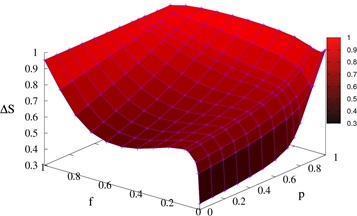Figure 1: Behavior of information entropy for the stochastic process labeled Professor. Typical values of the entropy variation are plotted as a function of the interaction parameter f and of the probability p in the ranges 0 f 1 and 0 p 1, respectively.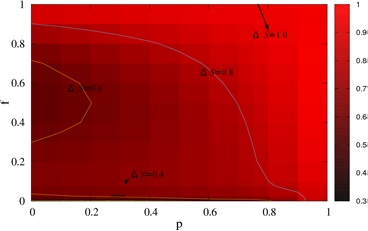Figure 2: Color map for the quantitative values of the information entropy, obtained from the data of Figure 1, arranged according to the parameters f and p, for the stochastic process labeled Professor. Contour curves are drawn in the range 0 ™ f ™ 1 and 0 ™ p ™ 1, to highlight regions of equal probability ∆S = 0.4, ∆S = 0.6, ∆S = 0.8 and ∆S = 1.0.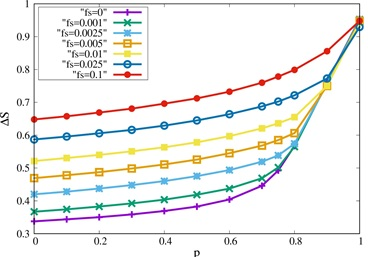Figure 3: We showed values of the entropy variation of Professor information for the ranges 0 ™ p ™ 1 and 0 ™ f ™ 0.1. The chosen probability of interaction values are f = 0, 0.001, 0.0025, 0.005, 0.01, 0.025 and 0.1, entropy grows in the sense that f and p grow.

Citation: Moura TRS (2018) Information Entropy for The Clinical Evaluation of Autism Spectrum Disorders. Res Adv Brain Disord Ther:  RABDT-106.  DOI: 10.29011/RABDT-106. 100006